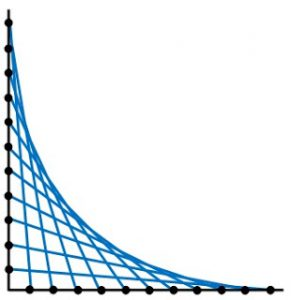1. ENVELOPE PATTERNS WITH STRAIGHT LINES

These straight lines form a family and the curves that you see, called envelopes, are tangent to each line in the family.

Draw two base lines and mark the same number of points at equal distances along each line. Join the outermost point on one base line to the innermost point on the other base line. Work your way inwards on one base line and outwards on the other base line joining corresponding points.

Experiment with different angles between the base lines, also using several pairs of base lines in the same diagram and using different colours.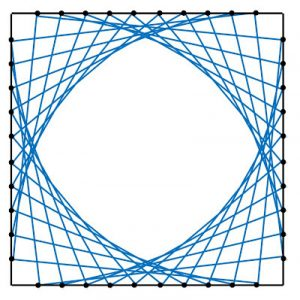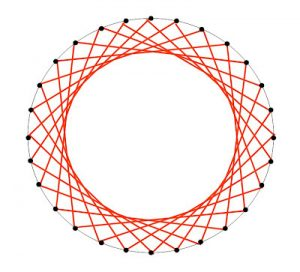Similar envelope patterns can be drawn by marking points equidistant around a circle. The envelope shown has N=28 points around the circle. It is drawn using the rule $n \to n + 7$ by joining each point to the 7th point around the circle, missing 6 points between them where n = 1, …, N.

2. PATTERNS WITH CIRCLES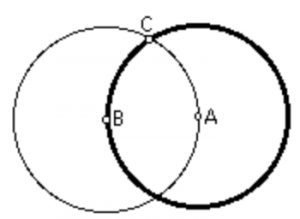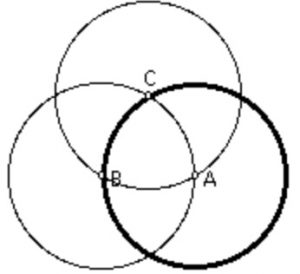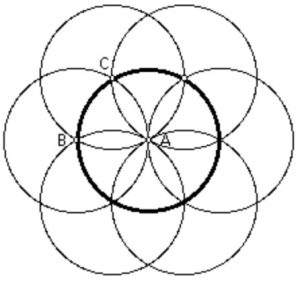a. Draw 2 circles with equal radii, intersecting at C, so that each goes through the centre of the other.

b. Draw a third circle of the same radius with centre C.

c. Draw 4 more circles with centres on the circle centre C and passing through A.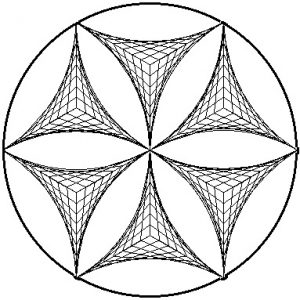Experiment with this basic construction and also by drawing only the arcs of the 6 outer circles that lie inside the circle centre A.

This design is a clever combination of the methods used in parts 1 and 2.

3. MORE PATTERNS FORMED BY STRAIGHT LINES

a.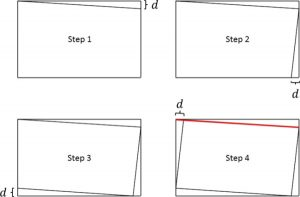Draw a rectangle, choose a small distance d and mark this distance along the edge of the rectangle as in Step 1. Mark the distance d along the next edge and draw the straight line as in Step 2.

b. Repeat this construction drawing straight lines spiralling inwards, marking the distance d along the next straight line that you will meet, as shown in Steps 3 and 4.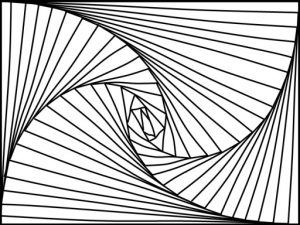c. Continue this construction drawing straight lines spiralling inwards, marking the distance d along the next straight line that you will meet and working your way towards the centre of the pattern.

Tagged with: